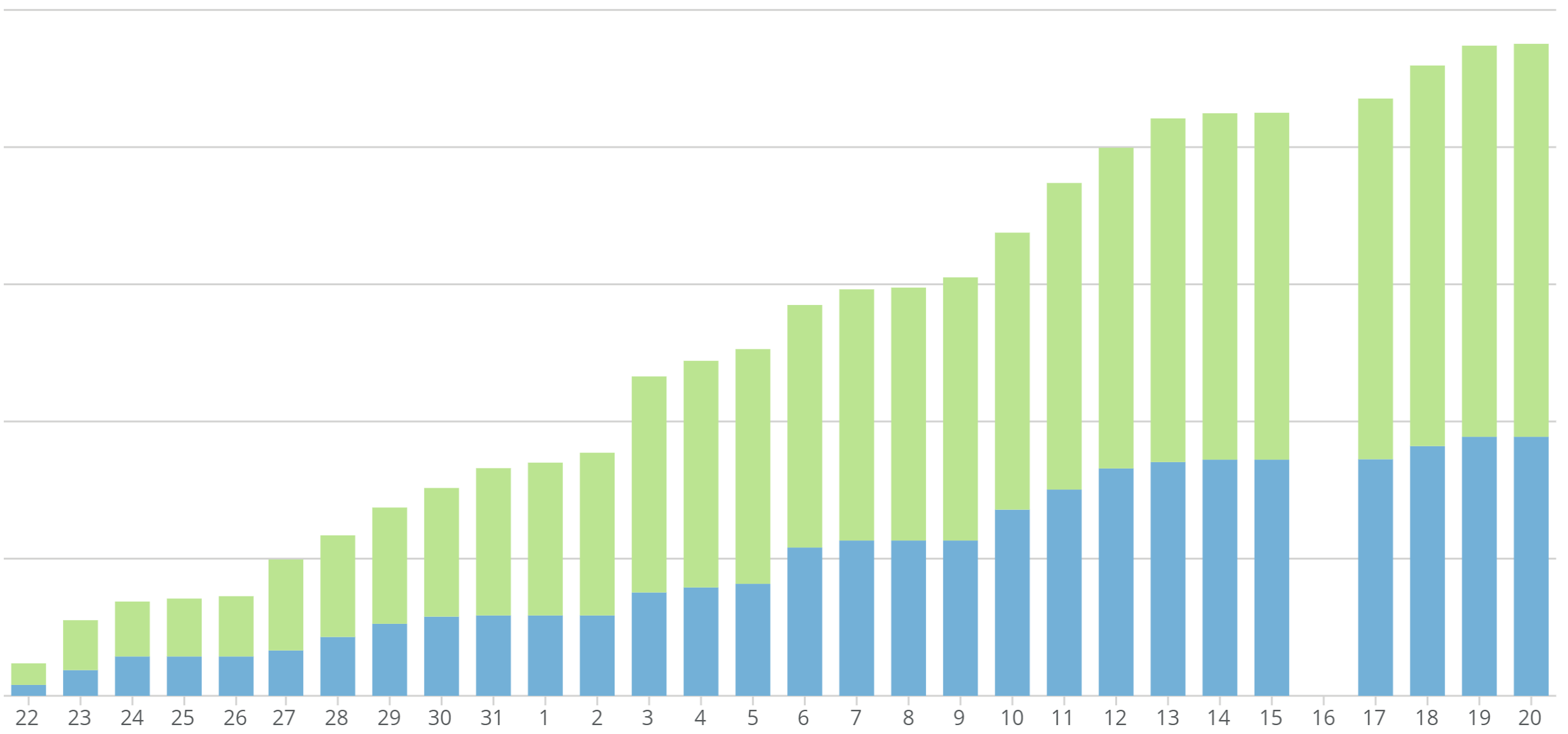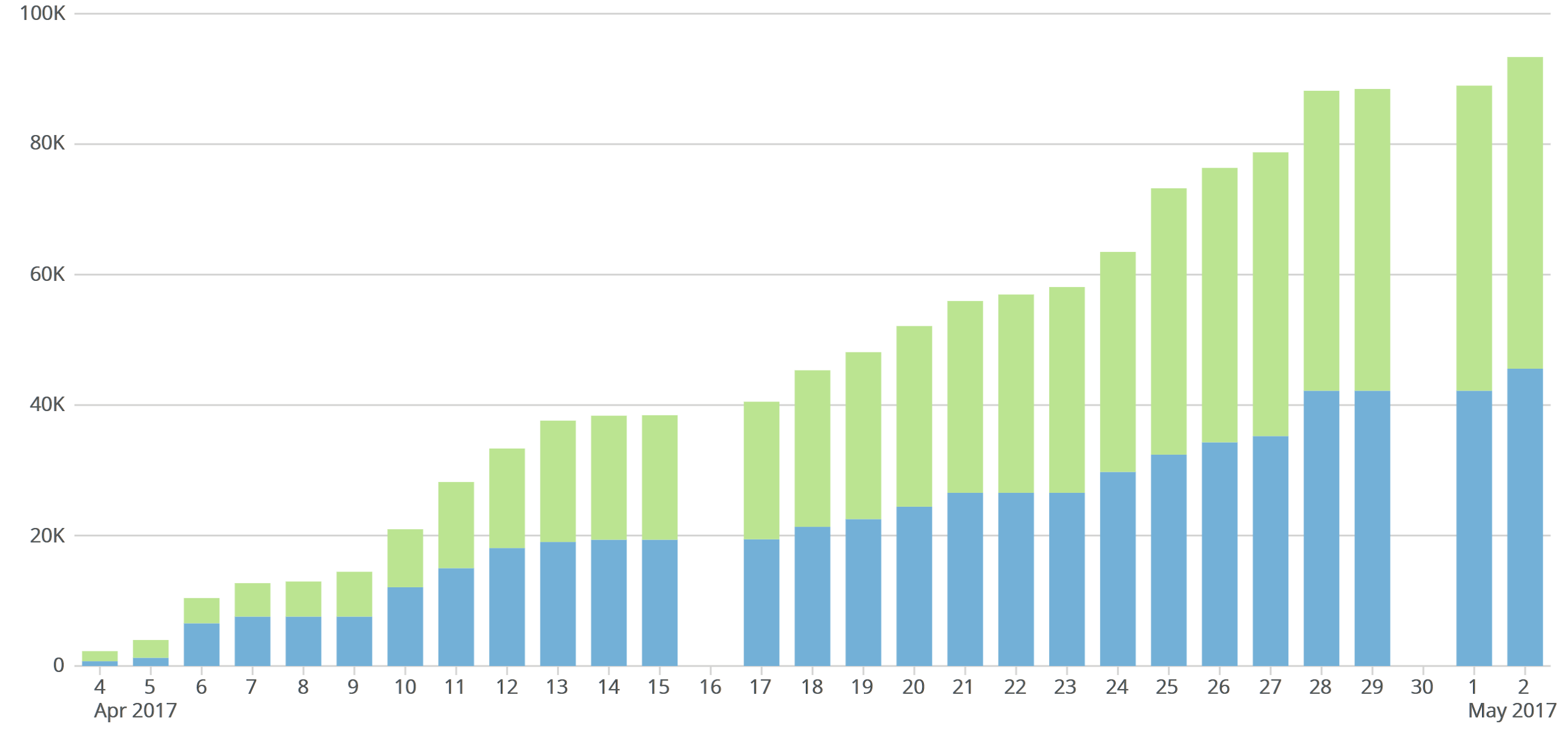# Bug?: Running Total zero value missing bar

Hi Dojo,

Has anyone else experianced the following?

On a running total card, if a period has no data to add to the total the bar is missing for that period.

Here is an example, we have a 30 day running total bar chart where on the 16th day no data exists to add to the total.

This should display the same bar value as the previous day, however given there is no data no bar is shown.I assume this is a bug somewhere in the calculations, am guessing logically the day value is retrived and added to the running total, however if the day value is zero or null the calculation breaks out of the function. might consider flipping this to get the running total first and then add the day value, or if null then 0, calculate.

Rgds,

K.

• Hi Dojo,

Is this issue being reviewed?

Now I've got a second missing bar, there should be no missing bars on a running total unless value is zero at intial previous day 30 and subsequent rolling zero days...Many thanks.

Rgds,

K.

• In case this is still an issue...You probably have null values...which Domo HATES!!!  Since it's a running total, just add a dummy set that adds a zero value for each period so that the thing has something to add to the previous total.  That may do the trick.

Hope all is well!

This discussion has been closed.